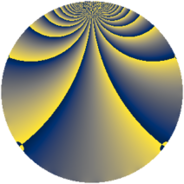# Properties

 Label 3920.1.btLevel $3920$ Weight $1$ Character orbit 3920.bt Rep. character $\chi_{3920}(79,\cdot)$ Character field $\Q(\zeta_{6})$ Dimension $20$ Newform subspaces $6$ Sturm bound $672$ Trace bound $11$

# Related objects

## Defining parameters

 Level: $$N$$ $$=$$ $$3920 = 2^{4} \cdot 5 \cdot 7^{2}$$ Weight: $$k$$ $$=$$ $$1$$ Character orbit: $$[\chi]$$ $$=$$ 3920.bt (of order $$6$$ and degree $$2$$) Character conductor: $$\operatorname{cond}(\chi)$$ $$=$$ $$140$$ Character field: $$\Q(\zeta_{6})$$ Newform subspaces: $$6$$ Sturm bound: $$672$$ Trace bound: $$11$$

## Dimensions

The following table gives the dimensions of various subspaces of $$M_{1}(3920, [\chi])$$.

Total New Old
Modular forms 184 20 164
Cusp forms 88 20 68
Eisenstein series 96 0 96

The following table gives the dimensions of subspaces with specified projective image type.

$$D_n$$ $$A_4$$ $$S_4$$ $$A_5$$
Dimension 20 0 0 0

## Trace form

 $$20q - 2q^{5} - 8q^{9} + O(q^{10})$$ $$20q - 2q^{5} - 8q^{9} + 2q^{25} + 4q^{29} - 4q^{41} - 4q^{45} + 2q^{61} - 12q^{69} - 10q^{81} - 2q^{89} + O(q^{100})$$

## Decomposition of $$S_{1}^{\mathrm{new}}(3920, [\chi])$$ into newform subspaces

Label Dim. $$A$$ Field Image CM RM Traces $q$-expansion
$$a_2$$ $$a_3$$ $$a_5$$ $$a_7$$
3920.1.bt.a $$2$$ $$1.956$$ $$\Q(\sqrt{-3})$$ $$D_{2}$$ $$\Q(\sqrt{-1})$$, $$\Q(\sqrt{-5})$$ $$\Q(\sqrt{5})$$ $$0$$ $$0$$ $$-1$$ $$0$$ $$q-\zeta_{6}q^{5}+\zeta_{6}q^{9}+\zeta_{6}^{2}q^{25}+q^{29}+\cdots$$
3920.1.bt.b $$2$$ $$1.956$$ $$\Q(\sqrt{-3})$$ $$D_{2}$$ $$\Q(\sqrt{-1})$$, $$\Q(\sqrt{-5})$$ $$\Q(\sqrt{5})$$ $$0$$ $$0$$ $$1$$ $$0$$ $$q+\zeta_{6}q^{5}+\zeta_{6}q^{9}+\zeta_{6}^{2}q^{25}+q^{29}+\cdots$$
3920.1.bt.c $$4$$ $$1.956$$ $$\Q(\zeta_{12})$$ $$D_{6}$$ $$\Q(\sqrt{-5})$$ None $$0$$ $$0$$ $$-2$$ $$0$$ $$q+(\zeta_{12}+\zeta_{12}^{3})q^{3}+\zeta_{12}^{4}q^{5}+(-1+\cdots)q^{9}+\cdots$$
3920.1.bt.d $$4$$ $$1.956$$ $$\Q(\zeta_{12})$$ $$D_{6}$$ $$\Q(\sqrt{-35})$$ None $$0$$ $$0$$ $$0$$ $$0$$ $$q+(\zeta_{12}+\zeta_{12}^{3})q^{3}+\zeta_{12}q^{5}+(-1+\cdots)q^{9}+\cdots$$
3920.1.bt.e $$4$$ $$1.956$$ $$\Q(\zeta_{12})$$ $$D_{6}$$ $$\Q(\sqrt{-35})$$ None $$0$$ $$0$$ $$0$$ $$0$$ $$q+(-\zeta_{12}-\zeta_{12}^{3})q^{3}+\zeta_{12}q^{5}+(-1+\cdots)q^{9}+\cdots$$
3920.1.bt.f $$4$$ $$1.956$$ $$\Q(\zeta_{12})$$ $$D_{2}$$ $$\Q(\sqrt{-1})$$, $$\Q(\sqrt{-35})$$ $$\Q(\sqrt{35})$$ $$0$$ $$0$$ $$0$$ $$0$$ $$q-\zeta_{12}q^{5}-\zeta_{12}^{4}q^{9}-\zeta_{12}^{3}q^{13}+\cdots$$

## Decomposition of $$S_{1}^{\mathrm{old}}(3920, [\chi])$$ into lower level spaces

$$S_{1}^{\mathrm{old}}(3920, [\chi]) \cong$$ $$S_{1}^{\mathrm{new}}(140, [\chi])$$$$^{\oplus 6}$$$$\oplus$$$$S_{1}^{\mathrm{new}}(560, [\chi])$$$$^{\oplus 2}$$$$\oplus$$$$S_{1}^{\mathrm{new}}(980, [\chi])$$$$^{\oplus 3}$$